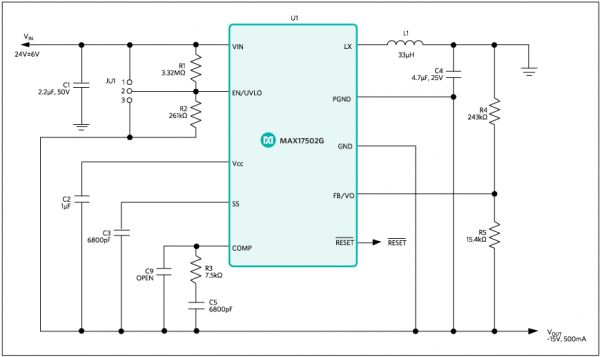# AppNote: How to Use the MAX17501 and MAX17502 for Negative Output Voltage Applications

Abstract: This article shows how to produce negative output voltages from positive input voltages using the MAX17501 and MAX17502 synchronous step-down converters.

## Introduction

Industrial control equipment such as programmable logic controllers, I/O modules, mass flow controllers, and various other sensors and supporting systems use analog components like amplifiers and multiplexers that operate on negative supply voltage. Typically operating at ±12V, ±18V or other variations, these voltages are generated from a 24V DC bus. Maxim’s portfolio of high-voltage synchronous buck regulators offer 50% lower power loss allowing customers to operate their equipment 50% cooler. In this application note, we discuss techniques to use these synchronous buck regulators to generate negative voltages.## Design Considerations

Synchronous buck converters can be configured to work in a buck-boost topology to produce negative output voltage from positive input voltage. This application note explains how the MAX17501 and MAX17502 synchronous step-down converters can be used to generate negative output voltage from positive input voltage. A -15V output voltage application is used to demonstrate the principle.

Table 1. Negative Output Voltage Power-Supply Requirements.

The sum of the maximum operating input voltage for the negative output application and the absolute value of the output voltage should not exceed the maximum operating voltage (60V) of the MAX17501 and MAX17502, as expressed by the following equation:
VIN_MAX + |VOUT| ≤ 60V
Therefore, for -15V output voltage, maximum operating input voltage can be as high as 45V. The minimum operating input voltage for the negative output voltage application should be greater than 4.5V.

### Calculating Duty Ratio

The expression for the duty ratio of the negative output power supply is shown below; ignoring the losses associated with the power switches and the inductor DC resistance: